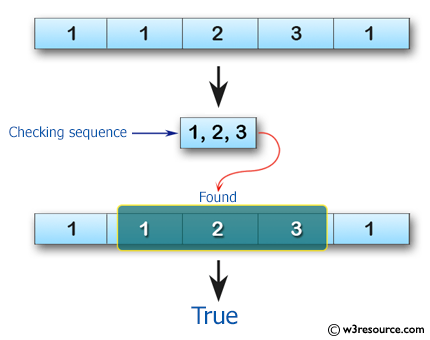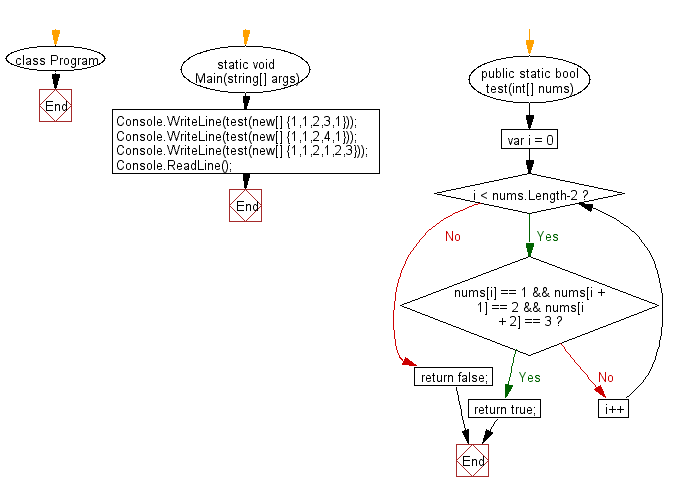﻿ C# - Check if a sequence of numbers appears in an array# C# Sharp Basic Algorithm Exercises: Check whether the sequence of numbers 1, 2, 3 appears in a given array of integers somewhere

## C# Sharp Basic Algorithm: Exercise-34 with Solution

Write a C# Sharp program to check whether the sequence of numbers 1, 2, 3 appears in a given array of integers somewhere.

Pictorial Presentation:Sample Solution:-

C# Sharp Code:

``````using System;
using System.Linq;

namespace exercises
{
class Program
{
static void Main(string[] args)
{
Console.WriteLine(test(new[] {1,1,2,3,1}));
Console.WriteLine(test(new[] {1,1,2,4,1}));
Console.WriteLine(test(new[] {1,1,2,1,2,3}));
}

public static bool test(int[] nums)
{
for (var i = 0; i < nums.Length-2; i++)
{
if (nums[i] == 1 && nums[i + 1] == 2 && nums[i + 2] == 3)
return true;
}
return false;
}
}
}
```
```

Sample Output:

```True
False
True```

Flowchart:C# Sharp Code Editor:

Improve this sample solution and post your code through Disqus

What is the difficulty level of this exercise?

Test your Programming skills with w3resource's quiz.

﻿